## 1.3.2 Introduction of a curve

There are two options to describe a curve which the mesh approximates:

• program a FORTRAN function, called FFRONT  of the following form:

FUNCTION FFRONT(I,X,Y)

where

I
is the reference number of the line (portion of the contour) described, and
X,Y
are the space variables.

A typical example of function FFRONT is given below:

```      FUNCTION FFRONT(I,X,Y)
IF ( I .EQ. 1) THEN
FFRONT = function(X,Y)
ELSE IF ( I .EQ. nnn )
FFRONT = ...
ELSE IF ...
...
END IF
END
```

• make use of the function parser :

A typical example of a function parser, for example the description of the line with reference 1 by a function, is the following:

COURBE01(X,Y) = X*X + (Y-1)*(Y-1) - .125

The first case corresponds to writing a FORTRAN function and linking it at execution. In the second case, the curves are given in a data file, thus avoiding performing a link.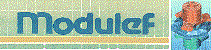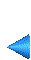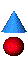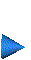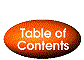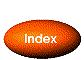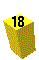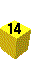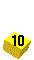Next: 1.3.3 Modification of the numbers Up: 1.3 Main ideas Prev: 1.3.1 The NOPO data structure Index Contents